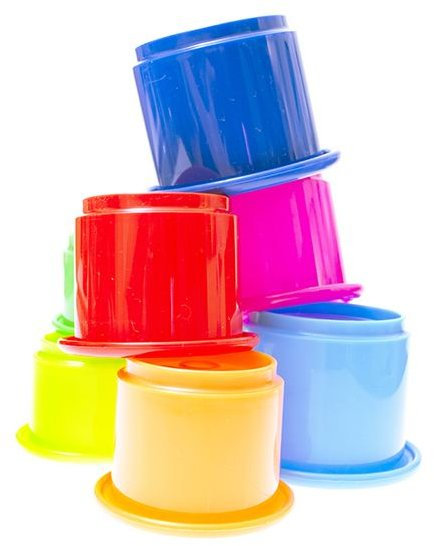# Middle School Geometry Lesson Plan on Relating Pi to the Circumference of a CircleObjective: Students will measure the circumference and diameter of circles to verify the value of pi and its role as a constant value in the relationship between circumference and diameter.

Materials: Paper, scissors, string, pencils, rulers, circular objects with flat tops or bottoms (such as cans, cake tins, water bottles, cups, round lids, etc.)

Note: Students should be familiar with locating the diameter of a circle prior to starting the lesson.

## Step One: Introduction

Provide a variety of circular objects with flat tops or bottoms for students to examine and handle. You may want to allow students to work in pairs or small groups for this activity.

Show students how to use their rulers to find the diameter of each object, and how to use string and rulers to measure each item’s circumference. Allow students to take a minimum of five objects back to their desks for their investigations.

## Step Two: Investigation and Data Collection

Ask students to measure and record the diameter and circumference of each object, noting the measurements of each dimension in a table (see sample table above).

Download the sample table.

## Step Three: Working Backward to Determine Role of Pi

After students have completed the measurement activity and filled in their tables, guide them in plugging the values of the measurements they recorded into the equation C/d=x, noting that

• C represents circumference,
• d represents diameter,
• and x represents the value of circumference divided by diameter.

Have your students fill in the equations, and work to solve the equations by dividing circumference by diameter for each item.

See the completed sample chart below.

Note: Values are rounded to the nearest hundredth.

## Step Four: Discussing the Results

Students should note that the solution to each equation should be (or close to, depending on values rounded to nearest hundredth) 3.14, the value of pi. Students should conclude that pi is a constant value in circles, which can be used when multiplied with diameter to find circumference of circles.

You can demonstrate a few examples of this relationship by multiplying diameter (as given by students from their charts) by pi and comparing with circumference as listed in their charts.

Assessment: Collect your students’ tables before providing them with a brief quiz (5 questions or less) to demonstrate that they are comfortable using measurements of diameter and circumference to verify the constant value of pi.

Extension: This lesson is a good introduction to using a formula to find the circumference of a circle.